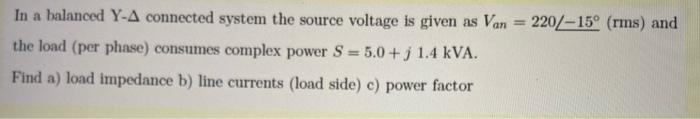# Question In a balanced Y-A connected system the source voltage is given as Van = 220/-15° (rms) and the load (per phase) consumes complex power S = 5.0+; 1.4 kVA. Find a) load impedance b) line currents (load side) c) power factor30QBND The Asker · Electrical EngineeringTranscribed Image Text: In a balanced Y-A connected system the source voltage is given as Van = 220/-15° (rms) and the load (per phase) consumes complex power S = 5.0+; 1.4 kVA. Find a) load impedance b) line currents (load side) c) power factor
More
Transcribed Image Text: In a balanced Y-A connected system the source voltage is given as Van = 220/-15° (rms) and the load (per phase) consumes complex power S = 5.0+; 1.4 kVA. Find a) load impedance b) line currents (load side) c) power factor